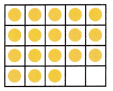# McGraw Hill Math Grade 1 Chapter 1 Lesson 4 Answer Key Counting and Writing from 16 to 20

All the solutions provided in McGraw Hill Math Grade 1 Answer Key PDF Chapter 1 Lesson 4 Counting and Writing from 16 to 20 as per the latest syllabus guidelines.

## McGraw-Hill Math Grade 1 Answer Key Chapter 1 Lesson 4 Counting and Writing from 16 to 20

Counting and Writing from 16 to 20

You can write numbers to tell how many.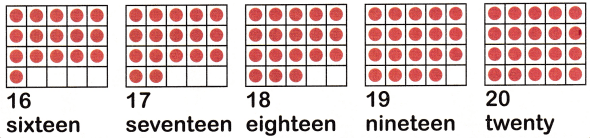Count

Tell how many. Circle the number.

Question 1.Answer: There are 16 circles in the above grid.

Question 2.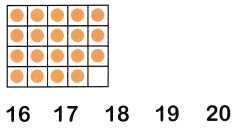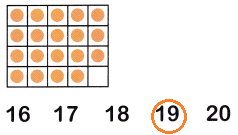There are 19 circles in the above grid.

Tell how many. Write the number.

Question 3.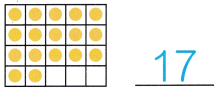There are 17 circles in the above grid.

Question 4.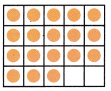_____
There are 18 circles in the above grid.

Question 5.________
There are 20 green circles in the above grid.

Question 6.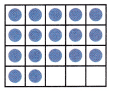________
There are 17 blue circles in the above grid.

Question 7._____